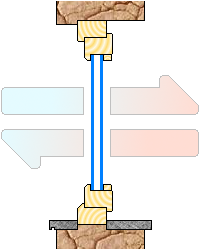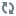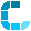# Calculation of the heat transfer coefficient and energy cost for fixtures.With this app you can calculate the heat transfer coefficient and the energy cost due to the losses of fixtures.

The heat transfer coefficient is the average heat flux which pass through a specific surface when there is a gradient of temperature, knowing the value of the heat transfer coefficient and the difference of temperature is possible to calculate the energy cost.

Frame data
Frame parameters.

Selection of the material

Specific heat transfer coefficientW/m2K

External visible width of the frame
in meters.
External visible height of the frame
in meters.

External dimension of the frame

W1 (m)

H1 (m)Parameters for glass and panels.

Selection type of panel

Heat transfer coefficient for the panel or glass.
Linear heat transfer coefficient of the
gap (only for multiple glass).

Specific heat transfer coefficientW/m2K

W/m K

Width of the panel
or glass in meters.
Height of the panel
or glass in meters.

Dimension of the panel

W (m)

H (m)

Number of equal panels

n.

Add a new panel
Delete last panel
Parameters for calculation of dispersions.

External temperature:

°C

Inner temperature:

°CResults Total surface m2 Area defined as frame m2 Area defined as panel m2 Heat transfer coefficient W/m2K Thermal power lost at the setted temperature kWh Thermal power lost (equivalent) at the setted temperature kcal Hourly consumption of methane of the frame at the setted temperature (normal cubic meter hour) Nm3/h

How much energy does this frame loose ?
If you already done the calculation, you can calculate how much does the door or the window cost annually with the characteristics that you have defined using the simple extra calculation below.

Annual cost with electric heating or electric air conditioning:
Cost = cost_kw x kWh x hours_day x days_month x months_year

Annual cost with heating using methane:
Cost = cost_m3 x Nm3/h x hours_day x days_month x months_year

Values cost_kw and cost_m3 must be read on the bill.

The hours per day, the days per month and months per year must be those when the temperatures are on average those setted.

Practical example with heating using methane:

• External door in softwood of 50mm, dimensions 0.8m x 2.1m
• 3 double glass (4-12-4), dimensions 0.18m x 1.6m
• Average temperatures = external -8°C, inner 21°C
• Methane cost per m3 = 0.88 Euro al m3
• Hourly cost = 0.0157 m3/h
• Calculation : 0.88 x 0.0157 x 10 x 30 x 4 = almost 17 Euro per year.

Notes:
The values in the list for linear heat transfer coefficient of the gaps for glass are average values, there are of different kind, you can insert also the value manually.

The temperature scales are wider than necessary for calculate industrial application as ovens, refrigerators or climatic chambers in general.

The calculation method can be extended in agreement with the basic norm: UNI EN 10077-1

Instructions
Want to help us improve ?
Notices